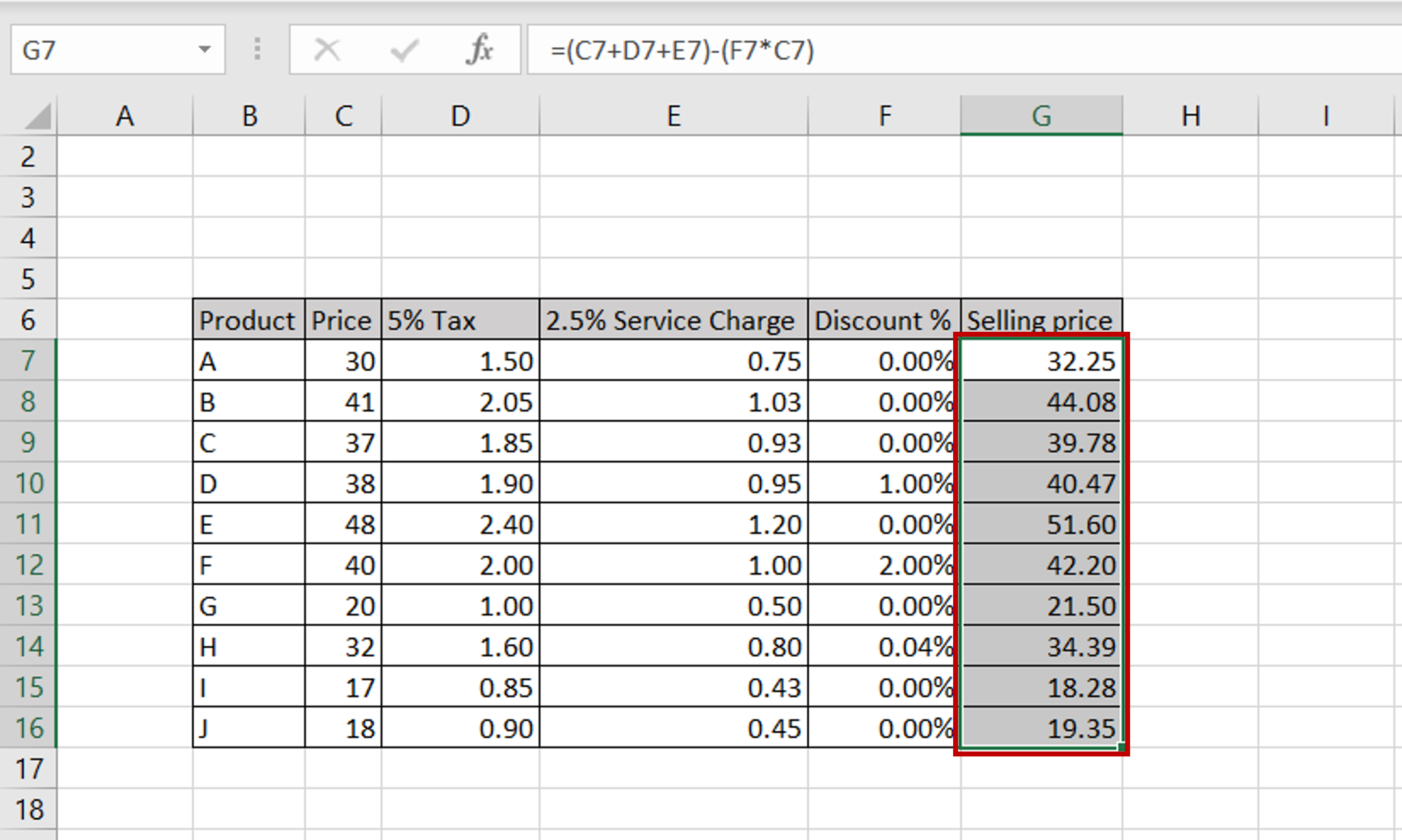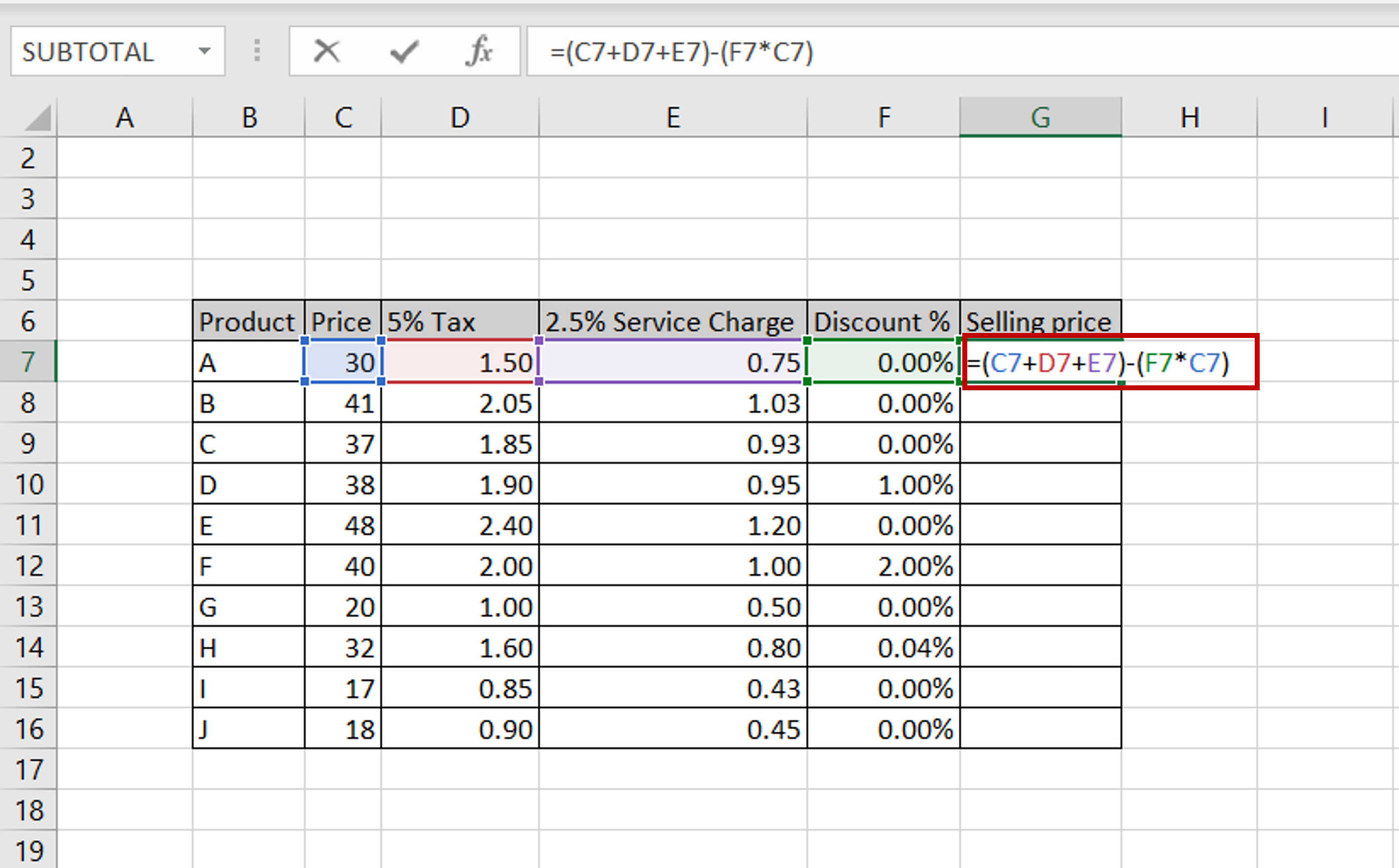# How to create a formula for multiple cells in Excel

You can watch a video tutorial here.One of the many great things about Excel is the ease with which complex formulas and calculations can be done. Here we will see how you can create a formula that contains values from multiple cells.

### Step 1 – Type the formula– In this example, we add tax and service charges to the product price, remove the discount, if any, and arrive at the final selling price
– In the destination cell type the formula using the cell references:
=(Price + 5% Tax + 2.5% Service Charge) – (Discount % * Price)

### Step 2 – Copy the formula– Using the fill handle from the first cell, drag the formula to the remaining cells
OR
a) Select the cell with the formula and press Ctrl+C or choose Copy from the context menu (right-click)
b) Select the rest of the cells in the column and press Ctrl+V or choose Paste from the context menu (right-click)